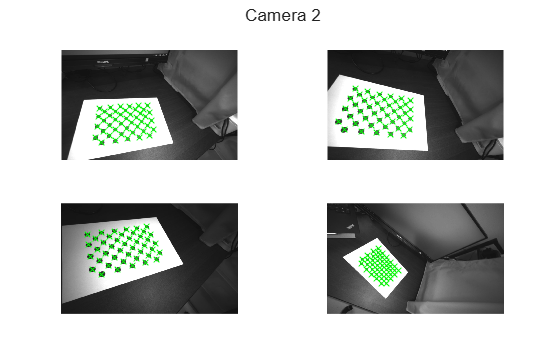# detectCircleGridPoints

Detect circle grid pattern in images

## Syntax

``imagePoints = detectCircleGridPoints(I,patternDims)``
``[imagePoints,imagesUsed] = detectCircleGridPoints(imageFileNames,patternDims)``
``[___] = detectCircleGridPoints(images,patternDims)``
``[imagePoints,pairsUsed] = detectCircleGridPoints(imageFileNames1,imageFileNames2,patternDims)``
``[___] = detectCircleGridPoints(images1,images2,patternDims)``
``[___] = detectCircleGridPoints(___,Name=Value)``

## Description

### Single Image Circle Grid Detection

example

````imagePoints = detectCircleGridPoints(I,patternDims)` detects a circle grid in a 2-D truecolor or grayscale image, `I`. For more details on circle grid patterns, see Circle Grid Patterns.```
````[imagePoints,imagesUsed] = detectCircleGridPoints(imageFileNames,patternDims)` detects a circle grid in the image files specified by `imageFileNames`, and additionally returns the list of images in which the circle grid is detected `imagesUsed`.```
````[___] = detectCircleGridPoints(images,patternDims)` detects a circle grid in the specified images `images`.```

### Stereo Pair Circle Grid Detection

example

````[imagePoints,pairsUsed] = detectCircleGridPoints(imageFileNames1,imageFileNames2,patternDims)` detects a circle grid in the stereo pairs of image files specified by `imageFileNames1` and `imageFileNames2`. The function additionally returns a list of the pairs in which the pattern is detected `pairsUsed`.```
````[___] = detectCircleGridPoints(images1,images2,patternDims)` detects a circle grid in the stereo pairs of image files specified by `images1` and `images2`.```

### Optional Arguments

````[___] = detectCircleGridPoints(___,Name=Value)` specifies options using one or more name-value arguments in addition to any combination of arguments from previous syntaxes. For example, `detectCircleGridPoints`(`I`,`patternDims`,`PatternType="symmetric"`) detects a symmetric circle grid in the specified workspace.```

## Examples

collapse all

Load an image containing a circle grid pattern into the workspace.

```imageFileName = fullfile(toolboxdir('vision'),'visiondata', ... 'calibration','circleGrid','mono','image08.jpg'); I = imread(imageFileName);```

Define the dimensions for the circle grid pattern.

`patternDims = [8 11];`

Detect the circle grid points.

`imagePoints = detectCircleGridPoints(I,patternDims,PatternType="symmetric")`
```imagePoints = 88×2 395.1729 358.4451 383.5573 388.4410 370.8857 420.9698 356.9887 456.5418 341.6686 495.7388 325.0617 538.9151 306.5055 586.8763 285.8323 640.1285 436.4195 363.5016 426.5806 393.4896 ⋮ ```

Display the detected points on the image.

```J = insertText(I,imagePoints,1:size(imagePoints,1)); J = insertMarker(J,imagePoints,'x',Color="green",Size=5); imshow(J) title("Detected a Circle Grid of Dimensions " + mat2str(patternDims))``````imageDir = fullfile(toolboxdir('vision'),'visiondata', ... 'calibration','circleGrid','stereo'); leftImages = imageDatastore(fullfile(imageDir,'left')); rightImages = imageDatastore(fullfile(imageDir,'right')); images1 = leftImages.Files; images2 = rightImages.Files;```

Define the dimensions for the circle grid pattern.

`patternDims = [4 11];`

Detect the circle grid points in the images.

`[imagePoints,pairsUsed] = detectCircleGridPoints(images1,images2,patternDims);`

Display the points from the first four images of camera one.

```figure t1 = tiledlayout(2,2,TileSpacing="compact",Padding="compact"); for i = 1:4 nexttile imshow(images1{i}) hold on plot(imagePoints(:,1,i,1),imagePoints(:,2,i,1),"gx"); end title(t1,"Camera 1")```Display points from the first four images from camera two.

```images2 = images2(pairsUsed); figure t2 = tiledlayout(2,2,TileSpacing="compact",Padding="compact"); for i = 1:4 nexttile imshow(images2{i}) hold on plot(imagePoints(:,1,i,2),imagePoints(:,2,i,2),"gx"); end title(t2,"Camera 2")```## Input Arguments

collapse all

Pattern dimensions, specified as a two-element vector that represents the number of circles in the x and y dimensions of the image, respectively. For more details on circle grid patterns, see Circle Grid Patterns.

AsymmetricSymmetricImage, specified as a 2-D truecolor image or grayscale image.

Data Types: `uint8` | `int16` | `uint16` | `single` | `double`

Images, specified as an H-by-W-by-numColorChannels-by-numImages array, where H and W describe the height and width of each image, respectively. numColorChannels is the number of color channels in each image, and numImages is the number of images.

Data Types: `uint8` | `int16` | `uint16` | `single` | `double`

Stereo pair images from camera one, specified as an H-by-W-by-numColorChannels-by-numImages array, where H and W describe the height and width of each image, respectively. numColorChannels is the number of color channels in each image, and numImages is the number of images

Data Types: `uint8` | `int16` | `uint16` | `single` | `double`

Stereo pair images from camera two, specified as an H-by-W-by-numColorChannels-by-numImages array, where H and W describe the height and width of each image, respectively. numColorChannels is the number of color channels in each image, and numImages is the number of images

Data Types: `uint8` | `int16` | `uint16` | `single` | `double`

Image file names, specified as a cell array of character vectors or an array of strings.

Stereo image file names for camera one, specified as a cell array of character vectors or an array of strings.

Stereo image file names for camera two, specified as a cell array of character vectors or an array of strings.

### Name-Value Arguments

Example: `detectCircleGridPoints`(`I`,`patternDims`,`PatternType="symmetric"`) detects a symmetric circle grid in the specified workspace.

Specify optional pairs of arguments as `Name1=Value1,...,NameN=ValueN`, where `Name` is the argument name and `Value` is the corresponding value. Name-value arguments must appear after other arguments, but the order of the pairs does not matter.

Circle grid pattern type, specified as `"asymmetric"` or `"symmetric"`.

Circle color, specified as `"black"` or `"white"`. Choose the color for the circle that has the strongest contrast with the background.

## Output Arguments

collapse all

Center coordinates of detected circle grid, returned as an M-by-2 matrix or an M-by-2-by-numPairs-by-2 array. M is the number of circle grids detected, calculated as the product of the number of circle grids detected in each dimension. Each row of the matrix or array specifies the x-y coordinates of the center of a circle grid.

• For single images — This argument is an M-by-2 matrix when detecting circle grids in single images.

• For stereo pairs — This argument is an M-by-2-by-numPairs-by-2 array when detecting circle grids in stereo pairs of images. numPairs is the number of image pairs in which a circle grid is detected. `imagePoints``(:,:,:,1)` returns the points from the first set of images, and `imagePoints``(:,:,:,2)` are the points from the second set.

Pattern detection flag, returned as a logical vector. A value of `true` in the `imagesUsed` vector indicates that the pattern has been detected in the corresponding image.

Stereo pair pattern detection flag, returned as a logical vector. A value of `true` in the `pairsUsed` vector indicates that the pattern has been detected in the corresponding image pair.

## Algorithms

For a circle grid pattern to be detected, the pattern must:

• Contain at least 16 circles

• Be fully visible in the image of a single camera or in both images of a pair of stereo cameras

## Version History

Introduced in R2021b